# Test: Heat Exchanger Level - 2

## 10 Questions MCQ Test Heat Transfer | Test: Heat Exchanger Level - 2

Description
Attempt Test: Heat Exchanger Level - 2 | 10 questions in 30 minutes | Mock test for Chemical Engineering preparation | Free important questions MCQ to study Heat Transfer for Chemical Engineering Exam | Download free PDF with solutions
QUESTION: 1

### In a counter flow heat exchanger, the working parameters for hot fluid are as follows: heat capacity = 2 kJ/kg K, mass flow inlet temperature 150oC , outlet temperature 100oC. For the cold fluid, the parameters are: heat capacity = 4 kJ/kg K, - mass flow rate 10 kg/s, and inlet temperature is = 20oC. The outlet temperature of the cold fluid in oC is

Solution: From steady state flow energy equation

mhcph(Thi - The) = mccpc(Tce - Tci)

∴ 5 x 2000(150 -100) = 10 x 4000(Tce - 20)

Tce = 32.5oC

QUESTION: 2

### Cold water at 10oC enters a counter flow heat exchanger at a rate of 8 kg/s , where it is heated by a hot water that enters the heat exchanger at 70oC and flows at a rate of 2kh/s . Assume specific heat of water to be cp = 4.18 kJ/kgoC . Determine the maximum theoretical heat transfer rate?

Solution:

Given mh = 2 kg/s

Mc = 8 kg/s

cph = cpc = 4.18kj/kgo-C

∴ mhcph 2 x 4.18 = 8.36 kW/oC

mhcph 8 x 4.18 = 33.4 kW/oC

∴ mhcph < mccpc

For maximum heat exchanger rate,

Qmax = (mcp)min(Thi - Tci)

⇒ Qmax = 8.36(70-10)

⇒ Qmax = 501.6 kW

QUESTION: 3

### A cross flow type air heater has an area of 50 m2. The overall transfer coefficient 100 W/m2 -and heat capacity of both hot and cold streams is 1000 W/K. The value of NTU is

Solution:

By definition

NTU = UA/(mcp)min

In the given situation, both capacity rate (mcp) of both hot and cold fluid are equal

∴ NTU = 100 x 50/1000 = 5

QUESTION: 4

The overall heat transfer coefficient for a shell and tube heat exchanger for clean surfaces is Uo = 400 W/m2- K. The fouling factor after one year of operation is found to be hfo = 200 W/m2 -K. The overall heat transfer coefficient at this time is

Solution:

Given Uo = 400 W/m2- K

Fouling factor, hFO = 200 W/m2-K

∴ New overall heat transfer coefficient can be predicted as follows

1/U = 1/U+1/hFO

1/U = 1/400 + 1/200

U = 33.33 W/m2-K

QUESTION: 5

In a solar assisted air conditioning system, 1 kg/sec of ambient air is to be preheated by the same amount of air leaving the system. A counter-flow heat exchanger having an area of 60m2 with overall heat transfer coefficient of 25 W/m2 - K is used for this purpose. Assuming for air is 1kJ/kg K. The effectiveness of the heat exchanger is

Solution: This is the case of a balanced counter flow heat exchanger.

∴ ε = NTU/1+NTU

Where NTU = UA/(mcp)min

For balanced heat exchanger,

(mcp)h = (mcp)c

∴ NTU = 60 x 25 / 1000 = 1.5

∴ ε = 1.5/1+1.5 = 0.6

QUESTION: 6

In a double-pipe heat exchanger, the cold fluid is water with inlet temperature of 20oC and mass flow rate of 20 kg/s and the hot fluid which also has water inlet temperature of 80oC and mass flow rate of 10 kg/s. For water cp = 4.2kj/kg oC.

. What is the maximum temperature to which the cold fluid can be heated in a parallel flow and in a counter flow heat exchanger?

Solution: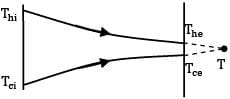For parallel flow heat exchanger maximum temperature of cold fluid will be reached when both hot and cold fluid exit at the same temperature.

Thus by steady state flow energy equation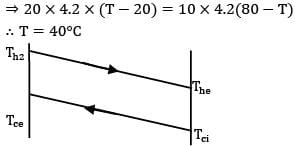⇒ 20 x 4.2 x (T - 20) = 10 x 4.2 (80-T)

∴ T = 40oC

For counter flow heat exchanger, maximum exit temperature of cold fluid is achieved when effectiveness of the heat exchanger is 1

i.e.

ε = 1

i.e.

(mccpc)(Tco - Tci)/(mccpc)(Thi - Tci) =1

1 = 20 x 4.2 x [T - 20]/10 x 4.2 x [80 - 20]

T = 50oC

QUESTION: 7

In a double-pipe counter flow heat exchanger 1000kh/h, oil having a specific heat 20 j/kg -K is cooled from 150oC to 125oC by 1250 kg/h of fluid having specific heat 16j/kg-K. The cold fluid leaves the heat exchanger at 75oC . In this case, the temperature at which the cooling fluid enters the heat exchanger and LMTD are __________

Solution: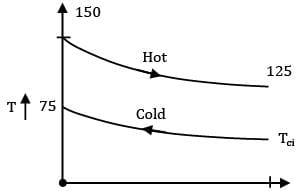x = 0 x x = l

mhcph = 1000 x 20 = 20,000j/hr-K

mccph = 1200 x 16 = 20,000j/hr-K

mhcph = mccph

Hence for a balanced Counter flow heat exchangers

ΔTm = ΔTi =ΔTe

∴ ΔTm = 150 -75 - 75oC

Thi - Tce = The - Tci

Tci - The = Tce - Thi

Tci = 50oC

*Answer can only contain numeric values
QUESTION: 8

A counter flow shell and tube type heat exchanger is used to heat water with hot exhaust gases. The water (c = 4180 j/kg-K ) flows at the rate of 2 kg/s and the exhaust gases (c = 1000 j/kg - K) flow at the rate of 5 kg/s. If the heat transfer surface area is 32 m2 and the overall heat transfer coefficient is 200 W/m2 -K , the effectiveness of the heat exchanger is __________.

(B) 0.63

Solution: Hot fluid [Exhaust gases]

CH = mHcH

∴ CH = 1000 x 5 = 5000 Watt/ ...①

Cold fluid [Water]

Cc = mccc

∴ Cc = 4180 x = 8360 Watt/K ...②

From ① & ②

CH < />c

∴ Cmin = CH = 5000 Watt/K

By definition,

C = Cmin/Cmax,

C = C CH/CC

C = 5000/8360

= 0.598

& NTU = UA/min

∴ NTU = 32 x 200/ 5000

= 1.28

For counterflow heat exchanger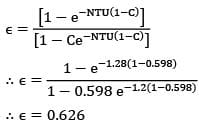∴ ε = 0.626

QUESTION: 9

In a counter flow heat exchanger, hot gases enter the system at and leave at . The temperature of the cold air entering the unit is . Its temperature at the exit is . The heat exchanger has an effectiveness of

Solution: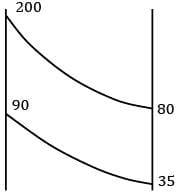Ch(Thi - The) = Cc(Tce - The)

Ch/Cc = Tce - Tci/Thi - The

Ch/Cc = 90-35/200-80 = 55/120

Ch < />C

∴ ε = Ch(Thi - The)/Ch(Thi - Tci)

ε = 200-80/200-35

ε = 0.7272

*Answer can only contain numeric values
QUESTION: 10

A coaxial tube counter flow heat exchanger is used to cool 0.03 kg/s of benzene from 360 K to 310 K with a counter flow of 0.02 kg/s of water initially at . If the inner tube outside diameter is 2 cm and the overall heat transfer coefficient based on outside area is 650 , determine the required length of the exchanger. Take the specific heats of benzene and water as 1880 and 4175 J/kg‐K, respectively.

(B) 2.60

Solution: By energy balance,

Q = mhcp(Th1 - Th2) = mccc(Tc2 - Tc1)

Q = 0.03 x 1880(360 - 310)

= 0.02 x 1475(Tc2 - 290)

Q = 2820 w,Tc2 = 323.8 K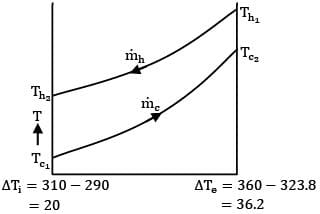ΔTi = 310 -290 ΔTe = 360 - 323.8

= 20 = 36.2

LMTD(ፀm) = 36.2 - 20/ In 36.2/20 = 27.3 K

Q = UoAom) = UoπdoL(θm)

L = 2820/650 x π x 0.02 x 27.3 = 2.53 mUse Code STAYHOME200 and get INR 200 additional OFF Use Coupon Code